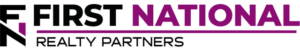# Using Discounted Cash Flow (DCF) Analysis to Evaluate Real Estate

### Key Takeaways

At the most fundamental level, a commercial real estate investment involves investing a sum of money today to purchase a stream of income in the future.  The concept itself is simple, but the hard part can be trying to determine how much to pay for that stream of income.

One of the most widely used, and often misunderstood, methods for valuing a stream of income is known as Discounted Cash Flow analysis.  It can be used to value an asset in any class, but is particularly relevant in commercial real estate transactions.

FNRP considers dozens of variables when performing a DCF analysis, which contributes to our track record of success. If you’re an accredited investor, click here to learn more about the commercial real estate investment opportunities we offer.

## What is Discounted Cash Flow Analysis?

The theory behind discounted cash flow analysis is known as the “time value of money,” which is the idea that a dollar today is worth more than a dollar in the future due to its ability to earn interest over time.  So, it follows that the value of an asset today is simply the sum of all future cash flows, discounted for the risk associated with earning them.

Mathematically, the formula for calculating an asset’s present value is: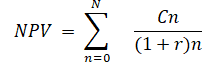If you are intimidated by this equation, don’t be.  The actual calculation is typically performed by using the “NPV” function in a spreadsheet with the following syntax:

NPV = (Discount Rate, Cash Flow 1, Cash Flow 2, Cash Flow 3, Cash Flow 4, Cash Flow 5,…)

No matter the method, three variables are needed to calculate net present value:  (1) cash flow per period; (2) the discount rate; and (3) the number of periods.  Let’s break them down individually.

## Cash Flows

A property’s cash flows are determined by forecasting them for a specific holding period and they can be broken down into two parts, recurring cash flows and the terminal cash flow.

The recurring cash flow forecast is known as a proforma and it incorporates the income and expenses associated with a given property.  Income is derived from tenant lease payments or unit sales and expenses are estimated by reviewing a combination of historic property performance and current market conditions.  The resulting before tax cash flow for each year in the holding period is the cash flow number used in the NPV spreadsheet function.

Because we are evaluating a property for investment purposes, it is implied that it will be sold at some point in the future.  The income earned from the sale is known as the “terminal cash flow” and it is calculated by taking the cash flow for the final year in the holding period and “capping it” using an estimate for the then market cap rate.  It can be the same as the discount rate.

### Discount Rate Real Estate

By definition, the discount rate is the return that an investor requires to take on the level of risk implied in the transaction.  In practice, choosing the discount rate in real estate is part art and part science.

The art of choosing a discount rate for a real estate investment is based on analysis of several variables that may be difficult to quantify including:  the likelihood of modeled cash flows actually occurring, property type, location, and the general economic conditions at the time of analysis.  Despite the number of variables, they all have one thing in common: risk.  The higher the perceived risk in the real estate transaction, the higher the return required by the investor, and thus the higher the discount rate.  The lower the perceived risk in a real estate investment, the lower the discount rate.

There are some generally accepted ranges within which the discount rate for real estate should fall; for most commercial real estate transactions, the discount rate should fall between 5% and 12%.  There is no definitive “right” or “wrong” discount rate, but the ultimate choice must be fully supported by data and previous transactions.

### Periods/Number of Periods

Discounted cash flow analysis is completed based on annual cash flows.  For an investment property, a “holding period” must be estimated and it is equivalent to the number of years that an investor plans to “hold” the property.  Because discounted cash flow analysis has a time component, the number of years in the holding period will have a material impact on the final result.

### Discounted Cash Flow Real Estate Evaluation Example

To illustrate how discounted cash flow analysis works, let’s go through two examples, a simple one and and a slightly more complex one.

#### A Simple Discounted Cash Flow Valuation Example

For the simple example, assume that we are considering a purchase of a property that produces \$10,000 in pre-tax cash flow per year.  Let’s also assume that our discount rate is 8.00% and that our holding period is 3 years.  Using these three variables, the net present value for this series of cash flows can be calculated using both of the methods described above:Regardless of the calculation method, the result is the same.  The net present value of these cash flows is \$25,771 and it represents the price an investor may be willing to pay for this stream of income if they require an annual return of 8.00%.

Perhaps this example is overly simplistic so let’s look at a slightly more complicated one.

#### A More Complex Discounted Cash Flow Valuation Example

Assume that the annual cash flows and the discount rate are the same at \$10,000 per year and 8.00% respectively.  But, this time, let’s assume that the cash flow in year 5 will be “capped” at the the discount rate of 8%, giving us a “terminal value.”  The cash flows are illustrated in the table below: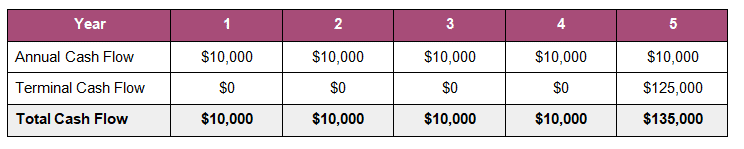The equation(s) for calculating the present value are the same, but with more variables: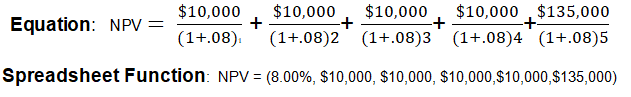Notice that the year 5 cash flow is the sum of the annual recurring cash flow and the terminal cash flow of \$125,000 (calculated as \$10,000/.08).

The net present value of this series of cash flows is \$125,000, inclusive of the sale in year five.  It is important to remember that the choice of a discount rate will have a material impact on the net present value of the same series of cash flows.

For example, one investor may look at this property and decide that it is more risky so they require a return of 9%.  In this case, the net present value is reduced to \$120,137.  Or, another investor may look at it and see less risk and they require an annual return of 6%, increasing the net present value to \$135,530.

The point is this, the discount rate drives the present value for a stream of cash flows coming from an investment property.  The higher it is, the lower the present value.  The lower it is, the higher the present value.  Choose wisely to avoid overpaying for a property.

### Interested In Learning More About Valuating Property Investment Opportunities?

In a DCF model, the cash flow forecast for each given year is based on the probability of it actually occurring.  In reality, the actual cash flows may differ materially from the forecast causing the investment outcome to under/over perform the initial forecast.

Forecasting cash flow is an imperfect science, but DCF is the most thorough method available to analyze the risk factors involved in a commercial real estate investment.  For our investments, we consider dozens of variables when performing DCF analysis and we believe this approach is a contributing factor to our track record of success.

First National Realty Partners is one of the country’s leading private equity commercial real estate investment firms. With an intentional focus on finding world-class, multi-tenanted assets well below intrinsic value, we seek to create superior long-term, risk-adjusted returns for our investors while creating strong economic assets for the communities we invest in.

Get instant access to all of our current and past commercial real estate deals.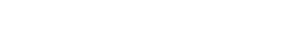## Subscribe Now

Get the latest news on real estate

1031 Exchange

1031 Exchange

### January 5, 2023

Real Estate Investment Types

## Get More From FNRP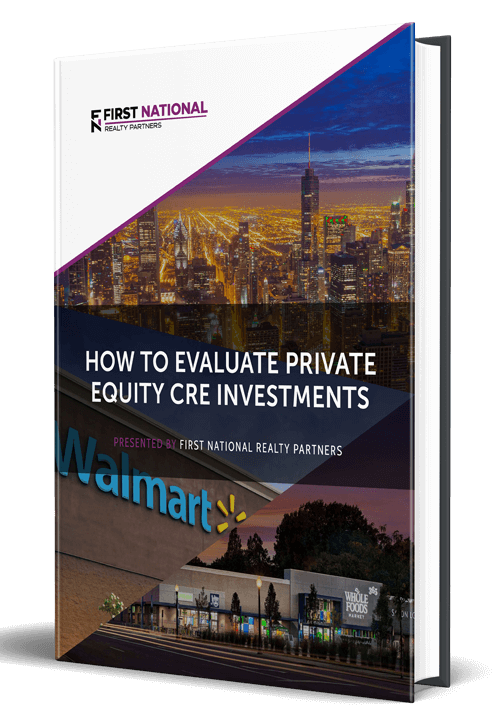## How to Evaluate Private Equity CRE Investments## How to Complete a 1031 Exchange with a Private Equity Sponsor